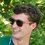# Prove that the last two digits are 28

Prove that if $n$ is an odd positive integer, then the last two digits of $2^{2n}(2^{2n+1}-1)$ in base $10$ are $28$.Note by Finn Hulse
7 years, 2 months ago

This discussion board is a place to discuss our Daily Challenges and the math and science related to those challenges. Explanations are more than just a solution — they should explain the steps and thinking strategies that you used to obtain the solution. Comments should further the discussion of math and science.

When posting on Brilliant:

• Use the emojis to react to an explanation, whether you're congratulating a job well done , or just really confused .
• Ask specific questions about the challenge or the steps in somebody's explanation. Well-posed questions can add a lot to the discussion, but posting "I don't understand!" doesn't help anyone.
• Try to contribute something new to the discussion, whether it is an extension, generalization or other idea related to the challenge.

MarkdownAppears as
*italics* or _italics_ italics
**bold** or __bold__ bold
- bulleted- list
• bulleted
• list
1. numbered2. list
1. numbered
2. list
Note: you must add a full line of space before and after lists for them to show up correctly
paragraph 1paragraph 2

paragraph 1

paragraph 2

[example link](https://brilliant.org)example link
> This is a quote
This is a quote
    # I indented these lines
# 4 spaces, and now they show
# up as a code block.

print "hello world"
# I indented these lines
# 4 spaces, and now they show
# up as a code block.

print "hello world"
MathAppears as
Remember to wrap math in $$ ... $$ or $ ... $ to ensure proper formatting.
2 \times 3 $2 \times 3$
2^{34} $2^{34}$
a_{i-1} $a_{i-1}$
\frac{2}{3} $\frac{2}{3}$
\sqrt{2} $\sqrt{2}$
\sum_{i=1}^3 $\sum_{i=1}^3$
\sin \theta $\sin \theta$
\boxed{123} $\boxed{123}$

Sort by:

Hint:Induction

- 7 years, 2 months ago

Okay... Can you explain?

- 7 years, 2 months ago

When n=1, the result is 28.Suppose for some n the condition is true.

Multiply by 16^2 and add $15.2^{2n+4}$ and you will get the next such number.So the new number will be congruent to

$256.28+15.2^{2n+4}\equiv 68+15.2^{2n+4}(mod100)$

But $15.2^{2n}$ for odd numbers n will be congruent to 60 modulo 100 (that's not hard to see), so we have proven in inductively.

- 7 years, 2 months ago

Oh, I guess so! Great job Bogdan. :D

- 7 years, 2 months ago

You probably have no idea how to pronounce my name :D

- 7 years, 2 months ago

Probably. How? :D

- 7 years, 2 months ago

Try using the speech feature on it.It sounds stupid :D

- 7 years, 2 months ago

HAHA yeah. But how is your name actually pronounced?

- 7 years, 2 months ago

I believe it should be pronounced like this: pronounce log, but with a B instead of an l, then say the name Dan :D

- 7 years, 2 months ago

Oh, that's what I thought. :D

- 7 years, 2 months ago